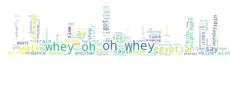Don't Look! I'm changing!

URL Copied

## Working with Wordclouds

This actually turned out to be rather straight forward

```import requests
from bs4 import BeautifulSoup as bs
from wordcloud import WordCloud, STOPWORDS
from PIL import Image #use to open the image
import matplotlib.pyplot as plt
import numpy as np
import os
%matplotlib inline```

A library exists to do what we want

`from wordcloud import ImageColorGenerator`

So we just get some text

```words =""
bs = bs(requests.get('http://www.myths.com/pub/lyrics/Bangles_.html').text, 'html.parser')
for x in bs.find_all("pre"): words = words+x.text
words = words.replace("\n", " ").lower() ```
`words`

Get an image and font face

`fontpath = os.path.join('EastSeaDokdo-Regular.ttf') `

Lets createa visualization function to do everything we could possibly want

```def show(img, s1, s2):
plt.figure(figsize=(s1,s2))
plt.clf() # Clear Figure
plt.imshow(img, interpolation='bilinear')
plt.axis('off')
plt.show()
#fig.set_figwidth(14) # set width
#fig.set_figheight(12) # set height ```

create an array mask of the image

```img = Image.open('510VP5aoo+L._AC_SL1402_.jpg')

and.... GO!

```cloud = WordCloud(stopwords=STOPWORDS, background_color='white', font_path=fontpath, max_words=300, width=300, height=200 ).generate(words)
show(cloud, 4, 4)```
`show(img, 4, 4)`
```cloud = WordCloud(mask= mask, stopwords=STOPWORDS, background_color='white', max_words=300, width=300, height=200 ).generate(words)
show(cloud, 4, 4)``````# max_font_size=1000
# font_path=fontpath,

Animating the SVG would be cool. But tricky. Animate the words within the svg?

Maybe next time.

For now, here are some miscellaneous notes on how to read files and do stuff with em. Like removing stop words.

```exClean = """
ds_2_words= ds_2.split()
print('Length before removing the stopwords: ', len(ds_2_words))
for word in ds_2_words:
if word in ENGLISH_STOP_WORDS:
ds_2_words.remove(word)
else:
pass

print('Length after removing the stopwords: ', len(ds_2_words))
"""```
```exeList = """
values = df['Client'].value_counts().keys().tolist()
counts = df['Client'].value_counts().tolist()
"""```
```exeRead = """
with open('word.txt', 'r') as f: #Read the data or words